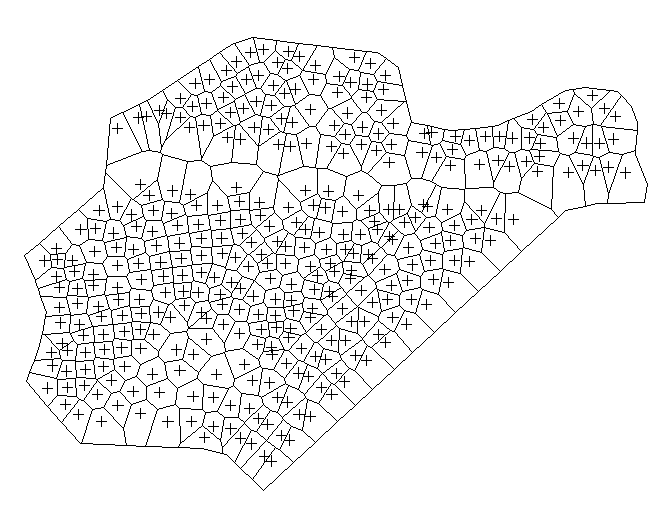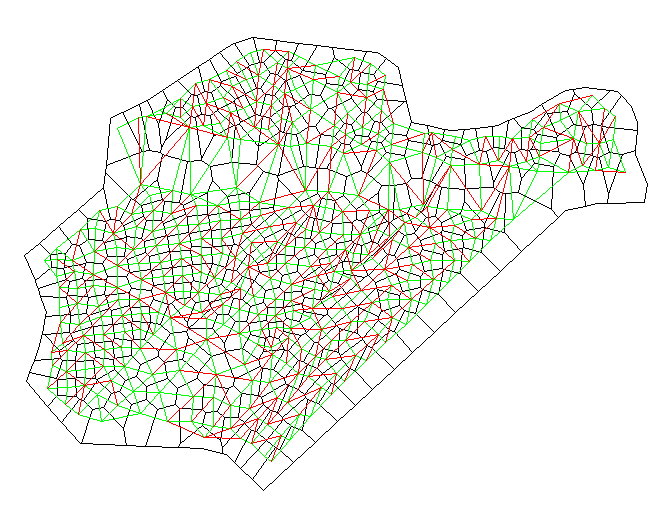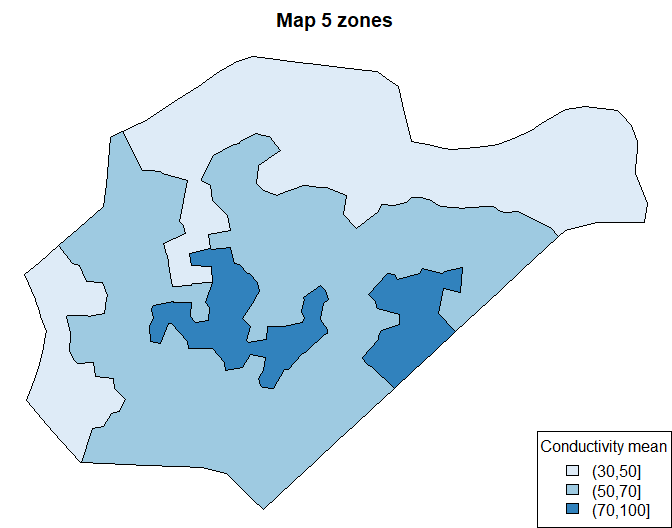# Zoning with GeoFIS

## Introduction

This vignette shows how to generate management zones from data with GeoFIS (Pedroso et al. 2010).

See Zoning documentation for more details about the zoning algorithm.

library(GeoFIS)

The zoning process accepts input dataset of class SpatialPointsDataFrame or SpatialMultiPointsDataFrame of the sp R package.
It keeps only the attributes that can be used in the zoning process, meaning numeric attributes, without missing values and with a range that is not limited to a unique value. The last condition is required by the min-max standardization process.
The input dataset must be in a projected coordinate reference system.

In this example the conductivity_2014 input dataset is used. It is included in the GeoFIS R package, each location is described by a single numeric attribute conduct:

data(conductivity_2014)
zoning <- NewZoning(conductivity_2014)

The zonable dataset used by the zoning process is available through the zonable_data method. In this example zonable_data is identical to conductivity_2014.

zonable_data <- zoning$zonable_data() ## Main parameters ### The border The border is a polygon used to limit the processed area. Only data points within the border are processed. If NULL the Convex Hull polygon of the input dataset will be used (computed with gConvexHull function of the rgeos R package). zoning$border <- NULL

An object of class SpatialPolygons can be used as border:

data(conductivity_border)
zoning$border <- conductivity_border #### Show the Voronoi diagram zoning$perform_voronoi()
vm <- zoning$voronoi_map() par(mar = c(0, 0, 0, 0)) plot(vm) plot(zoning$zonable_data(), add = TRUE)### The neighborhood

The neighborhood relation can be filtered by a minimal common edge length.

If NULL all contiguous Voronoi polygons are considered as neighbors:

zoning$neighborhood <- NULL The user can define the minimum edge length (in the same unit as the input dataset coordinate system) shared by two Voronoi polygons for being considered as neighbors: zoning$neighborhood <- 5

#### Show the neighborhood relations

The red lines shows the pairs of Voronoi polygons that are no longer considered as neighbors.

zoning$perform_neighborhood() nm <- zoning$neighborhood_map()
par(mar = c(0, 0, 0, 0))
plot(vm)
plot(subset(nm, filtered == FALSE), add = TRUE, col = "green")
plot(subset(nm, filtered == TRUE), add = TRUE, col = "red")### Attribute distance

This distance function is used for a given attribute. It computes the distance between two data points in the monodimensional attribute space. As many distance functions as there are attributes in the zonable dataset are needed (in a list if multiple attributes).
Two univariate distances are available: EuclideanDistance (the default) or FuzzyDistance. Or NULL if the attribute should not be used in the zoning process.

The fuzzy distance function is based on a fuzzy partition that allows for integrating expert knowledge into distance calculations (Guillaume, Charnomordic, and Loisel 2013; Guillaume and Charnomordic 2013). The partition must be a standardized fuzzy partition based on a FisIn object of the FisPro R package.

The following command-line sets the fuzzy distance based on 3 Mfs-partition defined by the following breakpoints: 20, 30, 100. The range of the conduct attribute is [12, 116]:

zoning$attribute_distance <- FuzzyDistance(NewFisIn(c(20, 30, 100), 12, 116)) The default value, used in this example, is the euclidean distance: zoning$attribute_distance <- EuclideanDistance()

### Zone distance aggregation

To compute the distance between two zones, all the data points included in the two zones are considered and the aggregation is done using the aggreg parameter:

$d(z_i,z_j) = (Aggreg) d(x,y), \forall x \in z_i, y \in z_j$ Three aggreg operators are available: MinimumDistance, MaximumDistance (the default) or MeanDistance.
The two zones to be merged at a given iteration are the ones for which the zone distance is minimum.

### Smallest zone

This criterion is used to determine the smallest size for a zone (number of points or area) to be kept in the final map. The zones with a size less than the threshold are included in the most compatible neighboring zone.
The two available parameters are: ZoneSize or ZoneArea.
The default value is ZoneSize with 1 point.

zoning$smallest_zone <- ZoneSize(1) ## Perform zoning zoning$perform_zoning()

Get the map with 5 zones:

map5 <- zoning$map(5) The result map contains for each zone: • the id • the number of points • the area • the mean of the attribute • the std of the attribute print(map5@data) #> id size area conduct_mean conduct_std #> 1 282 13 1691.965 93.15385 11.700402 #> 2 101 31 2442.451 87.29032 9.395241 #> 3 1 107 13123.461 36.40187 11.362226 #> 4 10 175 21098.067 60.05714 11.161011 #> 5 106 27 2683.125 41.77778 10.897955 Plot the map: library(RColorBrewer) palette <- brewer.pal(3, "Blues") breaks <- c(30, 50, 70, 100) colors <- palette[findInterval(map5$conduct_mean, vec = breaks)]
par(mar = c(0, 0, 2, 0))
plot(map5, col = colors, main = "Map 5 zones")
legend("bottomright", legend = levels(cut(map5\$conduct_mean, breaks)),
fill = palette, title = "Conductivity mean")### Save the map

Any map can be exported to various common file format, e.g. Shapefile: uncomment and replace tempdir() with the directory you want to export the map

# if (require(rgdal))
#   writeOGR(map5, dsn = tempdir(), layer = "map5", driver = "ESRI Shapefile")

## Publications

Guillaume, Serge, and Brigitte Charnomordic. 2013. “Fuzzy Partition-Based Distance Practical Use and Implementation.” In IEEE International Conference on Fuzzy Systems, Paper f-1136, edited by IEEE Catalog Number: CFP12FUZ-USB. Hyderabad, India: IEEE.
Guillaume, Serge, Brigitte Charnomordic, and Patrice Loisel. 2013. “Fuzzy Partitions: A Way to Integrate Expert Knowledge into Distance Calculations.” International Journal of Information Sciences 245: 76–95. https://doi.org/10.1016/j.ins.2012.07.045.
Leroux, Corentin, Hazaël Jones, Léo Pichon, Serge Guillaume, Julien Lamour, James Taylor, Olivier Naud, Thomas Crestey, Jean-Luc Lablee, and Bruno Tisseyre. 2018. “GeoFIS: An Open Source, Decision-Support Tool for Precision Agriculture Data.” Agriculture 8 (6). https://doi.org/10.3390/agriculture8060073.
Pedroso, Moacir, James Taylor, Bruno Tisseyre, Brigitte Charnomordic, and Serge Guillaume. 2010. “A Segmentation Algorithm for the Delineation of Management Zones.” Computer and Electronics in Agriculture 70 (1): 199–208. https://doi.org/10.1016/j.compag.2009.10.007.